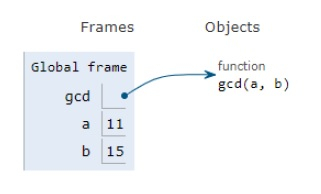# Python Program for Basic Euclidean algorithms

Problem statement− Given two numbers we need to calculate gcd of those two numbers and display them.

GCD Greatest Common Divisor of two numbers is the largest number that can divide both of them. Here we follow the euclidean approach to compute the gcd i.e. to repeatedly divide the numbers and stop when the remainder becomes zero.

Now let’s observe the solution in the implementation below −

## Example

Live Demo

# euclid algorithm for calculation of greatest common divisor
def gcd(a, b):
if a == 0 :
return b
return gcd(b%a, a)
a = 11
b = 15
print("gcd of ", a , "&" , b, " is = ", gcd(a, b))

## Output

gcd of 11 & 15 is = 1All the variables are declared in the local scope and their references are seen in the figure above.

## Conclusion

In this article, we have learned about how we can make a Python Program for Basic Euclidean algorithms.